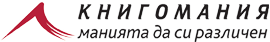Език
Валута

#Кошница

Няма наличност
ISBN
9780123825957
129,00 лв.
Advanced Engineering Mathematics provides comprehensive and contemporary...
Автор A.Jeffrey 1160 английски 2002 Academic Press 26842595 9780123825957 Енциклопедии и справочници
Advanced Engineering Mathematics provides comprehensive and contemporary coverage of key mathematical ideas, techniques, and their widespread applications, for students majoring in engineering, computer science, mathematics and physics. Using a wide range of examples throughout the book, Jeffrey illustrates how to construct simple mathematical models, how to apply mathematical reasoning to select a particular solution from a range of possible alternatives, and how to determine which solution has physical significance. Jeffrey includes material that is not found in works of a similar nature, such as the use of the matrix exponential when solving systems of ordinary differential equations. The text provides many detailed, worked examples following the introduction of each new idea, and large problem sets provide both routine practice, and, in many cases, greater challenge and insight for students. Most chapters end with a set of computer projects that require the use of any CAS (such as Maple or Mathematica) that reinforce ideas and provide insight into more advanced problems. A Student Solutions Manual is also available.
Напишете вашето мнение